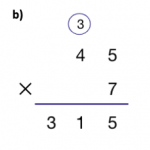# Algorithm in Math – Definition with Examples

Home » Math-Vocabluary » Algorithm in Math – Definition with Examples

## What Is an Algorithm?

An algorithm is a step-by-step process to solve a particular problem.

Think of it as a mathematical “recipe” to get to the bottom of a problem. If you follow the steps, you’ll be able to get to the answer in no time!

Example of an algorithm: A simple example of an algorithm you use every day is your morning routine. Say you get up at 6:30 a.m. to go to school. If you always get up, brush your teeth, drink water, go to the bathroom, and then have a bath, that can be the algorithm your body follows! Algorithms are all around us. It’s important to spot them to know how they function and later create our own.

There are a lot of procedures we apply in day-to-day life that we don’t realize are algorithms.

They include:

The daily timetable you follow in school

The rules to algebra formulae, like addition and subtraction

A technique you follow while playing sports

The rules to games you play with friends

## Algorithms in Math

Definition of Math Algorithm
An algorithm in math is a procedure, a description of a set of steps that can be used to solve a mathematical computation.
For example, a step-by-step procedure used in long divisions is a common example of a mathematical algorithm.

Example of Math Algorithm: The process of solving a mathematical problem such as, “What is 82 divided by 3?” could be achieved by doing the following algorithm:

How many times does 3 go into 8?

How many are left over now? 2

Put the 2 (tens) in front of the 3.

How many times does 3 go into 22?

The answer is 7, with a remainder of one.

And of course, the answer is 27 with a remainder of 1.

There are four simple steps for the standard algorithm for addition:

Step 1: Line up the numbers vertically by matching the place values.

Step 2: Add together the numbers that share the same place value, starting with the ones column.

Step 3: Write the sum below each column.

Step 4: If the sum of a column is greater than 9, carry over the tens digit to the next column.

### Standard Algorithm for Subtraction

There are four simple steps for the standard algorithm for addition:

Step 1: Line up the numbers vertically by matching the place values.

Step 2: Subtract the numbers that share the same place value, starting with the ones column.

Step 3: Write the difference below each column.

Step 4: If the number on the top in a column is less than the number at the bottom, then regroup before subtracting.

### Standard Algorithm for Multiplication

Algorithms are essential because of the large variety of applications in which they are used. Understanding how algorithms work is also crucial for developing problem-solving skills and building logical reasoning. Listed below are a few advantages of an algorithm:

• The algorithm creation process allows you to look at something in a rational and calculative manner, which helps greatly when it comes to solving different kinds of problems.
• Algorithms aid in bridging the communication gap, where following the procedure will help you reach the solution needed without going over the process repeatedly. You can follow the rules set up beforehand to reach the solution quicker.
• Algorithms use a definite procedure, and by using and optimizing them, people can solve problems much quicker.
• Algorithms are easy to debug because each step has its logical sequence.
• When we use algorithms, we can break down the problems into smaller steps or pieces, and therefore a programmer can easily convert them into an actual program.

Once you understand the foundation of algorithms, you will be able to create your own, saving yourself a lot of time. With SplashLearn’s fun activities and games, you can put your mathematical skills to the test and find algorithms in the questions you solve.

## Properties of Algorithms

Algorithms should be used to solve three objectives:

• Correctly execute a task: The job you want to do should be carried out with the intended results.
• Efficiently process the information given: Your system’s time and resources should be appropriately used to understand and later resolve the problem.
• Be easily understood: Algorithms are meant to make a job easier and, ideally, should be kept at a fundamental level of understanding.

Examples of algorithms in the real world

Many companies in the real world use algorithms to help their customers or grow their businesses and often try to improve those every day.

• YouTube: When you watch a few videos of a certain channel, you’ll notice that more and more videos by the same channel get recommended to you. It is due to YouTube’s recommendation algorithm, which collects information from your previous history to present videos of the same kind in your feed, so that you continue to watch videos on the platform.
• Social media: If you navigate to the ‘Explore’ page on Instagram, you’ll notice that many of the posts that show up are related to the ones you usually search for or like/comment on. The algorithm here identifies the posts you interact with and shows you more such posts because it believes that you enjoy such posts.
• Google: Google uses a very famous algorithm called PageRank to sort search results into an order that shows the most visited and authentic sites at the top. This algorithm considers dozens of parameters and provides the websites you would like to find fast.
• Lyft/Uber: Cab sharing companies like Lyft or Uber use positioning algorithms to help customers find vehicles close to them for an optimal experience. These global positioning algorithms also help drivers find the fastest routes to reach a certain destination. Algorithms also help such applications decide which customer they should pick or drop first.
• Facial recognition: Whenever companies require users to be verified, they can move past methods like user IDs and passwords to more secure authentications like facial recognition. Here, algorithms are used to identify a person and check if they have access to the things they want to get to.

## Tips to Master Algorithms

You can master algorithms by learning how to spot them in your day-to-day life. After that, you can break down the algorithm into bite-sized steps. You might have to test it a few times to notice a pattern in the way something takes place, but once you find it, you’ll be able to spot it again and again.

Try to figure out why each step in a process takes place. Once you understand why something happens, you’ll be able to connect the dots easier and figure out the logical sequence of events. In addition, it comes in very handy for figuring out how to create algorithms for yourself. With the help of the fun activities and games on SplashLearn, you’ll be able to master the basics of algorithms in no time!

## Solved Examples

Example 1: Write down the steps for making peanut butter and jelly sandwich.

Answer: Steps for making a peanut butter and jelly sandwich:
Step 1: Take 2 slices of bread.
Step 2: Apply peanut butter on one side of a slice.
Step 3: Apply jelly on one side of the other slice.
Step 4: Press both the slices of bread together.

Example 2: Write down the steps for the standard algorithm of subtraction.

Answer: The standard algorithm for subtraction follows these 4 steps:
Step 1: Line up the numbers vertically by matching the place values.
Step 2: Subtract the numbers that share the same place value, starting with the ones column.
Step 3: Write the difference below each column.
Step 4: If the number on the top in a column is less than the number at the bottom, then regroup before subtracting.

Example 3: Write an algorithm to know whether a number is odd or even.

Answer: The algorithm to find whether a number is odd or even:
Step 1: Divide the number by 2.
Step 2: If the number is completely divisible by 2, it is even, else it is odd.

Example 4: Write an algorithm to find the area of a rectangle.

Answer: The algorithm to find the area of the rectangle:
Step 1: Record the length of the shorter side as ‘b’.
Step 2: Record the length of the longer side as ‘l’.
Step 3: Area of a rectangle will be the product of ‘l’ and ‘b’.

## Practice Problems

1

### Which sequence will give the correct picture of an owl?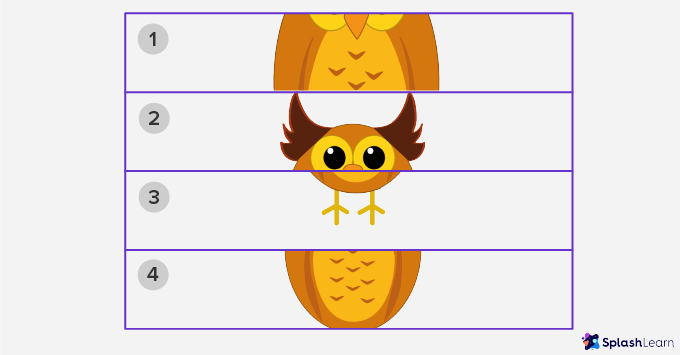2,4,1,3
2,1,4,3
2,1,3,4
2,4,1,3
CorrectIncorrect
The given sequence arranges the owl in the correct sequence.
2

### Which sequence will give the correct algorithm for boiling water? 1. Heat the pot until water boils 2. Turn the stove on 3. Take an empty pot 4. Put the water-filled pot on the fire 5. Pour water in empty pot

2,3,5,4,1
3,5,4,2,1
3,5,2,4,1
2,3,4,5,1
CorrectIncorrect
The given sequence gives the correct algorithm for boiling water.
3

### What will be the first step of addition algorithm

Placing digits vertically in columns
Carry over digits of a column
CorrectIncorrect
Correct answer is: Placing digits vertically in columns
The first step in adding any numbers is to arrange them vertically in columns.
4

### Which of the following correctly multiplies 45 ✕ 7 using the standard algorithm?

A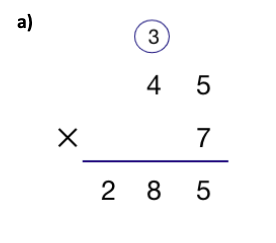B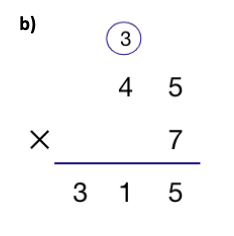C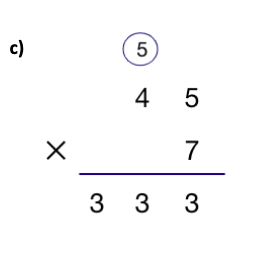D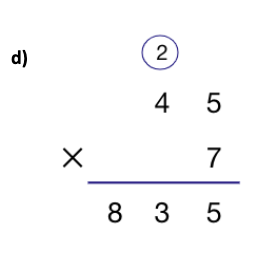CorrectIncorrect